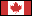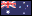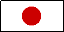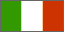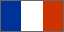## Search This Site## Browse by Vehicle Make and Model## Browse All Parts

Electric Scooter Repair Help > Conversion Calculator

# Millimeters to Inches Conversion CalculatorMillimeters to Inches Conversion CalculatorCalculator Converts Millimeters To Inch Decimals convert inches to millimetersMillimeters Inches =Inch Fraction to Inch Decimal Conversion ChartInch Fraction Inch Decimal
 1/16" = .0625"
 1/8" = .125"
 3/16" = .1875"
 1/4" = .25"
 5/16" = .3125"
 3/8" = .375"
 7/16" = .4375"
 1/2" = .5"
 9/16" = .5625"
 5/8" = .625"
 11/16" = .6875"
 3/4" = .75"
 13/16" = .8125"
 7/8" = .875"
 15/16" = .9375"

 This conversion calculator produces results in inch decimals and not inch fractions. Use the conversion chart above to convert inch decimals into inch fractions if necessary for your conversion.

## 1-800-908-8082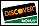## "When you need Electric Scooter Parts, go to: ElectricScooterParts.com"

We Ship Internationally